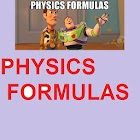Physics Formulas Pro

All Android applications categories

All Android games categories# Physics Formulas Pro

by: 1 10

10 Users
rating

## Screenshots

Description

No ads & swift & smooth app. You can try the free version before buying this one.

These are very useful Physics Formulas that serve as a quick reference.

With this application you can use your travel time to study, or just have it as a quick reference when needed.

This includes topics:
====================
Introduction to physics
----------------------------------
Relative deviation, Prefixes, Units for Area and Volume

Force and Motion
----------------------------
1. Average Speed, Velocity, Acceleration, Equation of linear motion
2. Finding velocity, Finding Acceleration, Graph of motion
3. Momentum, Newton's first law
4. Newton second and third law, Impulse, Gravitational field strength, Weight
5. Vertical Motion, Lifts
6. Lifts
7. Pulleys, Vectors
8. Inclined plane, Forces in equilibrium

Energy, Power and Work
------------------------------------
1.Work done
2. Energy, Power and efficiency

Pressure
--------------
1. Hooke's law
2. Density, Pressure, Gas Pressure
3. Pressure in a capillary tube, Barometer
4. Pascal's principle, Archimedes principle

Heat
--------
1. Heat, Boyle's law, Pressure law
2. Charles's law

Light
---------
1. Refractive index
2. Lens
3. Astronomical Telescope, Compound Microscope

Wave
----------
1. Wave, Interference
2. Oscillation, Displacement time and distance graph

Electricity
----------------
1. Sum of charge, Current
2. Potential difference,Resistance
3. Potential and potential difference
4. Electromotive force and internal resistance, Electrical Energy
5. Electrical Power, Efficiency
6. Electric Charges and fields
7. Capacitance
8. Current, Resistance
9. Inductive and RCL circuits
10. AC Circuits

Electromagnetism
--------------------------
1. Electromagnetism
2. Transformers
3. Magnetism

Electronics
----------------
Electronics, Cathode ray oscilloscope

Radioactivity
------------------

Useful for IIT JEE, AIEEE, etc.

It also has formulas for College Grade/Higher Grade Students/School Students.
The app is suitable for all levels of physics from high school to university and beyond.

Helps in all the following ways:
Physics, Physics Formulas, Physics Formula, Formulas Physics, Physics Formulae

from 1 reviews

"Awesome"

10